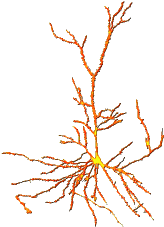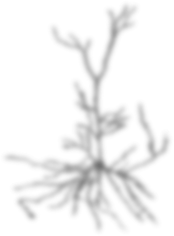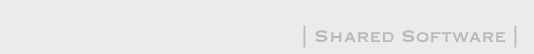Handedness Questionnaire

Instructions

For each of the activities below, please indicate:

Which hand you prefer for that activity?
Do you ever use the other hand for the activity?

Which hand do you prefer to use when:   Do you ever usethe other hand? Writing: no pref Left Right X Yes Left Right X Yes Left Right X Yes Left Right X Yes Left Right X Yes Left Right X Yes Left Right X Yes Left Right X Yes Left Right X Yes Left Right X Yes Left Right X Yes Left Right X Yes Left Right X Yes Left Right X Yes Left Right X Yes Evaluate

Laterality
Index (LI)
Decile
LI = −100
10th left
−100 ≤ LI < −92
9th left
−92 ≤ LI < −90
8th left
−90 ≤ LI < −87
7th left
−87 ≤ LI < −83
6th left
−83 ≤ LI < −76
5th left
−76 ≤ LI < −66
4th left
−66 ≤ LI < −54
3d left
−54 ≤ LI < −42
2d left
−42 ≤ LI < −28
1st left
−28 ≤ LI < 48
Middle
48 ≤ LI < 60
1st right
60 ≤ LI < 68
2d right
68 ≤ LI < 74
3d right
74 ≤ LI < 80
4th right
80 ≤ LI < 84
5th right
84 ≤ LI < 88
6th right
88 ≤ LI < 92
7th right
92 ≤ LI < 95
8th right
95 ≤ LI < 100
9th right
LI = 100
10th right

This handedness questionnaire was adapted from:

Oldfield, R.C. "The assessment and analysis of handedness: the Edinburgh inventory." Neuropsychologia. 9(1):97-113. 1971.

©2008 Mark S Cohen, Updated August 19, 2008

FAQ and HELP
We appreciate ideas to make brainmapping.org more useful. Submit your suggestions for changes and links here: [ suggestions ]